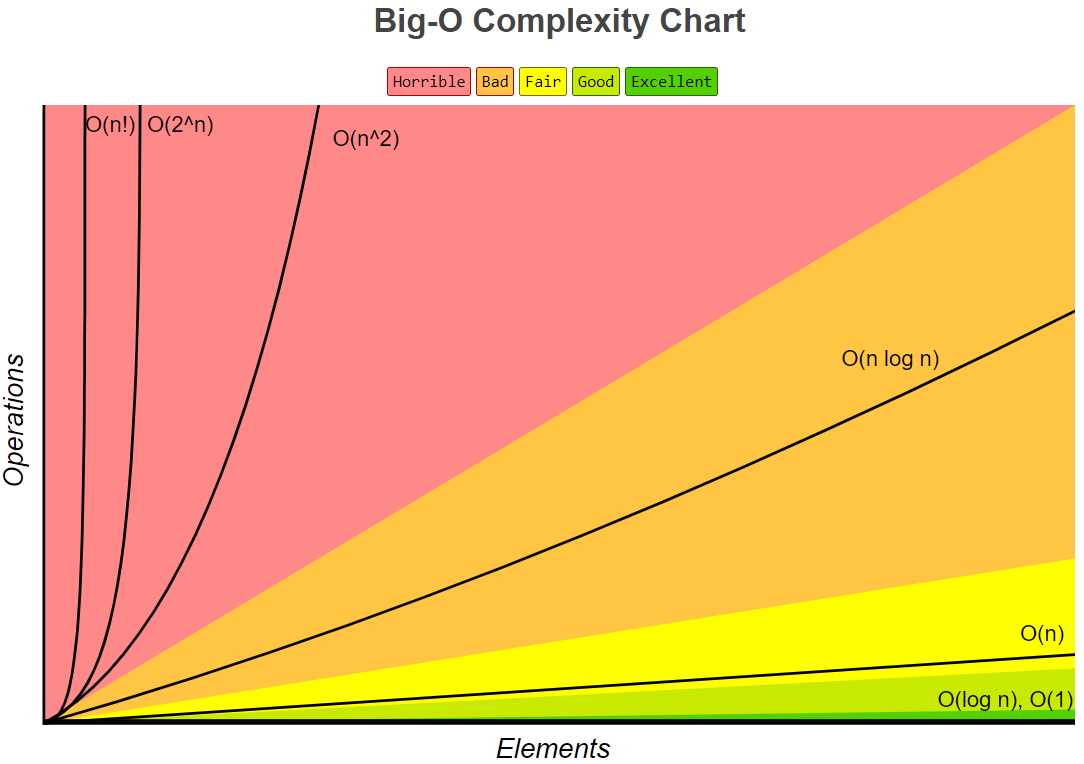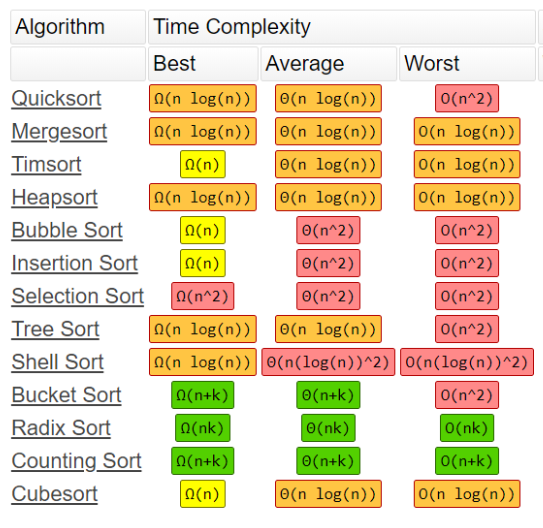# 【算法】算法的时间复杂度和空间复杂度

contents structure [-]

# 2.推导大O阶

• 用常数1取代运算中的所有加法常数
• 在修改后的运行次数中，只保留最高项
• 如果最高项阶存在且不为1，则去除与这个项相乘的参数

#include <stdio.h>

int main()
{
printf("Hello, World! \n");
printf("Hello, World! \n");
printf("Hello, World! \n");
printf("Hello, World! \n");
printf("Hello, World! \n");
printf("Hello, World! \n");

return 0;
}

    int n=100,sum=0;          //1
for(int i=0;i<n;i++){     //n
sum+=(i+1);
}
printf("%d",sum);         //1

    int n=100;
int sum=0;
sum=(1+n)*n/2;
printf("%d",sum);

    int i=1,n=100;          //1
while(i<n){             //x
i=i*2;
}
printf("%d",i);         //1

# 3.最优、平均、最差时间复杂度

def findElement(arr, ele):
length = len(arr)
for index in range(length):
if arr[index] == ele:
return index
return -1;

arr = [1,2,3,4]
target = 10
res = findElement(arr ,target)
print(res)

# 4. 常见算法的复杂度图表posted @ 2018-03-26 00:35  HDWK  阅读(587)  评论(0编辑  收藏  举报